# Ben and Siew's housing loan

Ben and Siew are considering buying an apartment at Southbank in Melbourne for a total cost of $700,000. They have$300,000 in the bank, and they want to borrow no more than 80% of the total cost. A major bank is offering housing loans at the standard variable rate of 7.05%. If they borrow their maximum amount, and repay monthly over a 25 year period, the monthly repayment is $3,975.84 per month as estimated by the Excel PMT function shown in Figure 1 (cell C9). PMT function syntax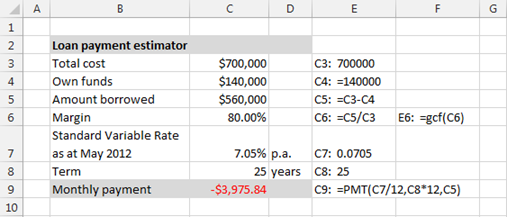Fig 1: Monthly loan repayment - cell C9 They are trying to resolve two issues. First, how much the monthly payment amount would be if they contribute more of their own funds to the purchase. Second, how much the monthly payment amount would vary if they decide on a loan over a different term. Using Excel cell formulae, Siew has set up the repayment table shown by table a in Figure 2.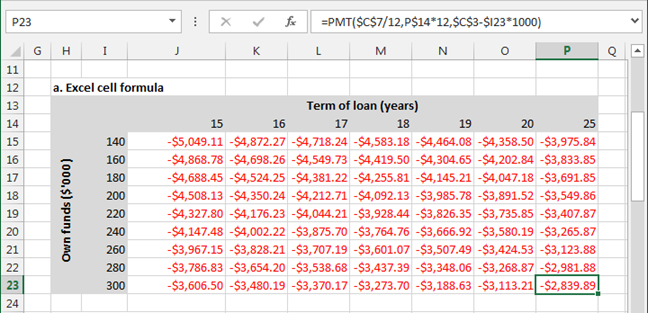Fig 2: Repayment table - using Excel cell formulae Required: answer each of the following questions. ### QUESTION a Excel cell formula: Refer to Figure 2 - If all of the formulae in range J15:P23 (the range with the repayment values), were originally a Copy and Paste from cell J15, what is the formula in cell J15? J15: =PMT($C$7/12,J$14*12,$C$3-$I15*1000) Note: to answer this question you need to identify the elements with relative referencing - shown in red.  P23: =PMT($C$7/12,P$14*12,$C$3-$I23*1000) J15: =PMT($C$7/12,J$14*12,$C$3-$I15*1000) ### QUESTION b Excel array formula: Suppose now that Siew has done a Copy and Paste of the table (a) in Figure 2, and she intends to enter array formula in the selection (J28:P36) shown in table (b) in Figure 3.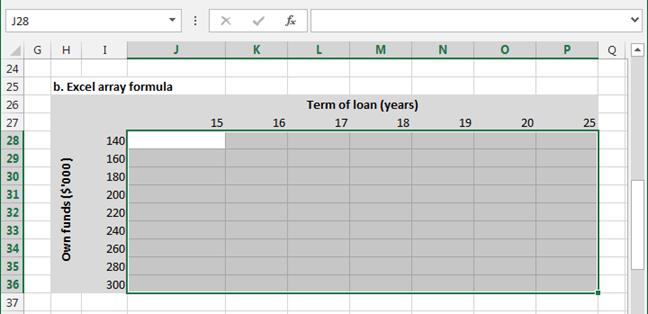Fig 3: Repayment table - using Excel array (CSE) formulae What array formula would she type in the formula bar? =PMT($C$7/12,J27:P27*12,$C$3-I28:I36*1000) Note: to answer this question you need to replace the cell references with vectors - shown in red.  P23: =PMT($C$7/12,P$14*12,$C$3-$I23*1000) J15: =PMT($C$7/12,J27:P27*12,$C$3-I28:I36*1000) How would she complete the formula? Just hit the Enter key? An array formula requires the key combination Ctrl+Shift+Enter to complete the entry List two important features or attributes of the array formula entered in the range J28:P36 1. Every cell in the array contains the same formula: =PMT($C$7/12,J27:P27*12,$C$3-I28:I36*1000) 2. For each cell in the array, the formula bar begins and ends with a curly brace (bracket) {}: In this case, {=PMT($C$7/12,J27:P27*12,$C$3-I28:I36*1000)}. The braces are not entered by the user, but are returned by the CSE process. 3. You cannot change part of the array. To edit or delete the array, you must select the entire array. ### QUESTION c Excel data table Suppose that Siew has done another Copy and Paste of the table (a) in Figure 2, and she has also added additional data as shown in table (c) in Figure 4. Column T now contains the amount borrowed. The amounts are in whole dollars, but formatted to show thousands. See the Get Cell Formula (gcf) in cells T56 and T57. This is the framework for a Data Table.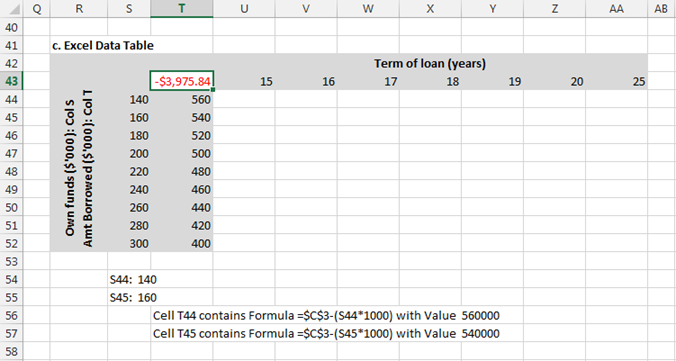Fig 4: Repayment table - using Excel Data Table with two inputs Note: the Data Table input cell (see figure 5) cannot contain a formula, and must use an absolute cell reference. Thus, the amount borrowed has been inserted in column T. What formula is required in cell T43 of the table in Figure 4, in order to set up the Data Table? =PMT(C7/12,C8*12,C5) Note: This is the same formula as shown in figure 1 - cell C9. How would you activate/display the Data Table dialog shown in Figure 5?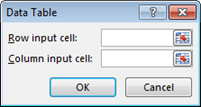Fig 5: Data Table dialog On the Excel ribbon: Data > What-If Analysis > Data Table ... What are the coordinates of the range (top left cell, bottom right cell), that must be selected before the Data Table is activated? The coordinates of the range: T43:AA52. In other words - Select the range of cells that contains the formula (T42), both the row and column of values (U43:AA43 and T44:T52), and the cells in which you want the calculated values (U44:AA52). What are the absolute cell references for the Row input cell, and the Column input cell, for the Data Table dialog in Figure 5. Row input cell:$C$8 Column input cell:$C\$5

Excel data validation: Excel data tables have a maximum of two dimensions. Siew is anxious to add a third dimension, by being able to easily change the annual interest rate. You suggest a validation rule be applied to cell C7. You decide on the following vector of rates: int = {0.0600, 0.0605, 0.0610, 0.0615, 0.0620, 0.0625, 0.0630, 0.0635, 0.0640, 0.0645, 0.0650, 0.0655, 0.0660, 0.0665, 0.0670, 0.0675, 0.0680, 0.0685, 0.0690, 0.0695, 0.0700, 0.0705, 0.0710, 0.0715, 0.0720, 0.0725, 0.0730}. The vector is stored in the Excel range (B3:B29) on Sheet2, named IntRate with workbook scope.

From the dropdown list of validation criteria shown in Figure 6, which item would Siew Allow to link to the vector of rates?

Allow: List

What would she enter for the chosen Allow item, Validation criteria. In other words, after she selects the Allow item, what would she do next?

After selecting List, enter the source property as Source: =IntRate

## Notes

PMT - Calculates the payment (PMT) for a loan based on constant payments and a constant interest rate. The loan is an annuity.
Syntax: PMT(rate,nper,pv,[fv],[type])
argument / parameterdetails
raterequired- the interest rate for the annuity.
nperrequired- the total number of periods or instalments for the annuity
pv required- the present value, or the total amount that a series of future payments is worth now.
fvoptional- the future value, or a cash balance you want to attain after the last payment is made. If fv is omitted, it is assumed to be 0 (zero), that is, the future value of the annuity is 0 - the loan has been repaid.
typeoptional- the number 0 (zero) or 1 and indicates when payments are due. 0 or omitted, end of period - an ordinary annuity; 1 beginning of period - an annuity due.

• This example was developed in Excel 2013 64 bit